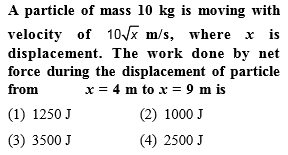You’ve reached the end of your free Videos limit.
#2 | Solved Problems
(Physics) > Work,Energy and Power
Related Practice Questions :Complete Question Bank + Test Series
Complete Question Bank

Difficulty Level:

A block of mass 50 kg slides over a horizontal distance of 1 m. If the coefficient of friction between their surfaces is 0.2, then work done against friction is

(1) 98 J

(2) 72J

(3) 56 J

(4) 34 J

Complete Question Bank + Test Series
Complete Question Bank

Difficulty Level:

A body of mass m is moving in a circle of radius r with a constant speed v. The force on the body is $\frac{m{v}^{2}}{r}$ and is directed towards the centre. What is the work done by this force in moving the body over half the circumference of the circle

(1) $\frac{m{v}^{2}}{\pi {r}^{2}}$

(2) Zero

(3) $\frac{m{v}^{2}}{{r}^{2}}$

(4) $\frac{\pi {r}^{2}}{m{v}^{2}}$

Complete Question Bank + Test Series
Complete Question Bank

Difficulty Level:

A man pushes a wall and fails to displace it. He does

(1) Negative work

(2) Positive but not maximum work

(3) No work at all

(4) Maximum work

Complete Question Bank + Test Series
Complete Question Bank

Difficulty Level:

A body moves a distance of 10 m along a straight line under the action of a force of 5 N. If the work done is 25 joules, the angle which the force makes with the direction of motion of the body is

(1) 0°

(2) 30°

(3) 60°

(4) 90°